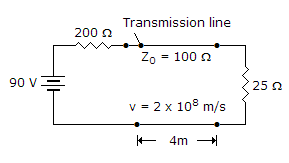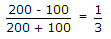# Electronics and Communication Engineering - Microwave Communication

### Exercise :: Microwave Communication - Section 1

36.

The action of backward wave oscillator is similar to that of

 A. klystron amplifier B. reflex klystron oscillator C. TWT D. magnetron

Explanation:

It is somewhat similar to TWT and can deliver microwave power over a wide frequency band.

It has an electron gun and a helix structure. However the interaction between electron beam and RF wave is different than in TWT.

The growing RF wave travels in opposite direction to the electron beam.

The frequency of wave can be changed by changing the voltage which controls the beam velocity.

Moreover the amplitude of oscillations can be decreased continuously to zero by changing the beam current.

It features are:

1. Frequency range - 1 GHz to 1000 GHz.

2. Power output - 10 mV to 150 mW (continuous wave) 250kW (pulsed).

It is used as signal source in transmitters and instruments.

37.

In the given figure the reflection coefficient at source end isA. 1 B. -1 C. 1/3 D. -1/3

Explanation:.

38.

Assertion (A): Artificial transmission lines are frequently used in laboratories.

Reason (R): An artificial transmission line can be used to represent an actual line and can also be used as a delay circuit, as attenuator, as filter network etc.

 A. Both A and R are correct and R is correct explanation of A B. Both A and R are correct but R is not correct explanation of A C. A is correct but R is wrong D. A is wrong but R is correct

Explanation:

Artificial transmission lines have many applications. One of the applications is to simulate an actual line in the laboratory.

39.

In a backward wave oscillator the wave travelling along the line winds itself back and forth

 A. True B. False

Explanation:

It is somewhat similar to TWT and can deliver microwave power over a wide frequency band.

It has an electron gun and a helix structure. However the interaction between electron beam and RF wave is different than in TWT.

The growing RF wave travels in opposite direction to the electron beam.

The frequency of wave can be changed by changing the voltage which controls the beam velocity.

Moreover the amplitude of oscillations can be decreased continuously to zero by changing the beam current.

It features are:

1. Frequency range - 1 GHz to 1000 GHz.

2. Power output - 10 mV to 150 mW (continuous wave) 250kW (pulsed).

It is used as signal source in transmitters and instruments.

40.

A line is excited by a 100 V dc source. If reflection coefficients at both ends are 1 each then

 A. there will be no oscillations on line B. there will be only 1 or 2 oscillations on line C. there will be a finite number of oscillations on line D. the oscillations will continue indefinitely